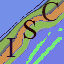Trending ▼   ResFinder# ISC Board Specimen 2011 : Mathematics

5 pages, 39 questions, 39 questions with responses, 100 total responses,00ISC 12th Indian School Certificate Examination (ISC), New Delhi
+Fave Message
 Home > isc >Formatting page ...

MATHEMATICS (Three hours) (Candidates are allowed additional 15 minutes for only reading the paper. They must not start writing during this time) ---------------------------------------------------------------------------------------------------------------------------Section A Answer Question 1 (Compulsory) and five other questions Section B and Section C Answer two questions from either section B or Section C All working, including rough work, should be done on the same sheet as and adjacent to, the rest of the answer The intended marks for questions or parts of questions are given in brackets [ ] Mathematical tables and graph papers are provided. Slide rule may be used. ------------------------------------------------------------------------------------------------------------------------------- SECTION A Question 1 x 1 3 (i) If is a symmetric matrix then find the value of x . 2x + 3 x + 2 (ii) If cos(2 sin 1 x ) = 1 find the values of x 9 (iii) Find the value of k if the line y = 4 x + k  touches the parabola y 2 = 16 x . (iv) Evaluate: Lim (1 + sin x )cot x x 0 (v) Evaluate: si n  xdx    2 (vi) Evaluate: 3 + 5 cos x log[ 3 + 5 sin x ]dx  0 (vii) Five books on Mathematics and 3 books on Physics are placed at random on a bookshelf. What is the probability that books on Mathematics are placed side by side on the bookshelf?  (viii) If the two lines of regression are 4 x + y = 225 and x + 4 y = 150 , find the value of Karl Pearson s coefficient of correlation for the data represented by x and y .  (ix) If ( 3 + i )6 = x + iy find the values ' x ' and y . (x) Solve log dy = 4x 2 y 2 . dx   ------------------------------------------------------------------------------------------------------------------------------1 ISC Specimen Question PaperFormatting page ...

Top Contributors
to this ResPaperluckykaul(17)Rutwik Pandya(17)Oraan (13)Anonymous(12)Formatting page ...Formatting page ...Formatting page ...Additional Info : ISC Board Specimen Question Paper 2011 Mathematics
Tags : ISC Board Specimen Question Paper 2011 Mathematics, Mathematics question paper, isc specimen Mathematics syllabus. , ISC Board, Council for the Indian School Certificate Examinations, ISC 2018 - 2019 Examination, 2019, 2018, 2017, 2016, 2015, 2014, 2013, 2012, 2011, ISC Solved Specimen Question Papers 2018 - 2019, ISC Solved Mock Guess Exam Papers, ISC Board Model Paper with Answers, Detailed Solutions & Explanations, isc sample question bank, past / old / last year online test papers, syllabus, online coaching.